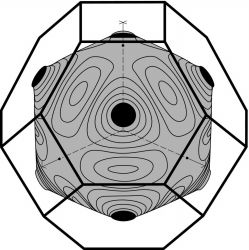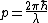# Fermi Surface

Also found in: Wikipedia.

## Fermi surface

The surface in the electronic wavenumber space of a metal that separates occupied from empty states. Every possible state of an electron in a metal can be specified by the three components of its momentum, or wavenumber. The name derives from the fact that half-integral spin particles, such as electrons, obey Fermi-Dirac statistics and at zero temperature fill all levels up to a maximum energy called the Fermi energy, with the remaining states empty. See Fermi-Dirac statistics

The fact that such a surface exists for any metal, and the first direct experimental determination of a Fermi surface (for copper) in 1957, were central to the development of the theory of metals. A surprise arising from the earliest determined Fermi surfaces was that many of the shapes were close to what would be expected if the electrons interacted only weakly with the crystalline lattice. The long-standing free-electron theory of metals was based upon this assumption, but most physicists regarded it as a serious oversimplification. See Free-electron theory of metalsFermi surface of copper, as determined in 1957; two shapes were found to be consistent with the original data, and the other, slightly more deformed version turned out to be correct

The momentum p of a free electron is related to the wavelength λ of the electronic wave by the equation below,where &planck; is Planck's constant divided by 2&pgr;. The ratio 2&pgr;/λ, taken as a vector in the direction of the momentum, is called the wavenumber k. If the electron did not interact with the metallic lattice, the energy would not depend upon the direction of k, and all constant-energy surfaces, including the Fermi surface, would be spherical.

The Fermi surface of copper was found to be distorted (see illustration) but was still a recognizable deformation of a sphere. The polyhedron surrounding the Fermi surface in the illustration is called the Brillouin zone. It consists of Bragg-reflection planes, the planes made up of the wavenumbers for which an electron can be diffracted by the periodic crystalline lattice. The square faces, for example, correspond to components of the wavenumber along one coordinate axis equal to 2&pgr;/a, where a is the cube edge for the copper lattice. For copper the electrons interact with the lattice so strongly that when the electron has a wavenumber near to the diffraction condition, its motion and energy are affected and the Fermi surface is correspondingly distorted. The Fermi surfaces of sodium and potassium, which also have one conducting electron per atom, are very close to a sphere. These alkali metals are therefore more nearly free-electron-like. See Brillouin zone

In transition metals there are electrons arising from atomic d levels, in addition to the free electrons, and the corresponding Fermi surfaces are more complex than those of the nearly free-electron metals. However, the Fermi surfaces exist and have been determined experimentally for essentially all elemental metals.

The motion of the electrons in a magnetic field provides the key to experimentally determining the Fermi surface shapes. The simplest method conceptually derives from ultrasonic attenuation. Sound waves of known wavelength pass through the metal and a magnetic field is adjusted, yielding fluctuations in the attenuation as the orbit sizes match the sound wavelength. This measures the diameter of the orbit and Fermi surface. The most precise method uses the de Haas-van Alphen effect, based upon the quantization of the electronic orbits in a magnetic field. Fluctuations in the magnetic susceptibility give a direct measure of the cross-sectional areas of the Fermi surface. See De Haas-van Alphen effect, Skin effect (electricity);, Ultrasonics

## Fermi Surface

a constant-energy surface in quasimomentum (p) space, separating the occupied states of electrons in a metal from states in which there are no electrons at T = 0°K. Electrons that lie on the Fermi surface and in a narrow region of quasimomentum space near the Fermi surface are responsible for most of the properties of metals. This is associated with the high density of conduction electrons in a metal; such electrons densely occupy the energy levels in the conduction band (seeDEGENERATE GAS and ). Every metal is characterized by its own Fermi surface, so that the shapes of the surfaces vary. For a free-electron gas, the Fermi surface is a sphere.

The volume ΩF bounded by Fermi surface—that is, the volume per unit cell in quasimomentum space—is determined by the density n of conduction electrons in a metal: 2ΩF/(2πℏ)3 = n. The average size of the Fermi surface for good metals is ~ ℏ/a, where is Planck’s constant and a is the lattice constant. Usually, n ≈ 1/a3. In most metals, small pockets whose volume is substantially less than (2π)3n/2 are found in addition to a large Fermi surface. The pockets determine many of the quantum properties of metals in a magnetic field, such as the de Haasvan Alphen effect. In semimetals, the volume bounded by the Fermi surface is small in comparison with the size of a unit cell in quasimomentum space.

If occupied states of electrons lie within a Fermi surface, the surface is called an electron surface; if empty states lie within a Fermi surface, the surface is called a hole surface. The two types of Fermi surfaces may coexist. For example, the Fermi surface for bismuth consists of three electron ellipsoids and one hole ellipsoid. The symmetry of crystals is reflected in Fermi surfaces. In particular, the surfaces are periodic with a period of 2πℏb, where b is an arbitrary reciprocal lattice vector. All Fermi surfaces have a center of symmetry. Fermi surfaces of complex topology, that is, multiply connected surfaces, are encountered; such a Fermi surface is simultaneously an electron surface and a hole surface. If a Fermi surface continuously penetrates all of quasimomentum space, the surface is said to be open. If a Fermi surface breaks up into pockets, each of which fits in one unit cell of quasimomentum space, the surface is said to be closed. For example, Li, Au, Cu, and Ag have open Fermi surfaces; K, Na, Rb, Cs, In, Bi, Sb, and Al have closed Fermi surfaces. Fermi surfaces sometimes consist of open and closed pockets. The velocities of electrons lying on the Fermi surface are vF ≈ 108 cm/sec. The direction of the vector v is normal to the Fermi surface.

The geometrical characteristics of Fermi surfaces, such as the shape, curvature, and cross-sectional area, are related to the physical properties of metals. These relationships make it possible to construct Fermi surfaces on the basis of experimental data. For example, the reluctance of a metal depends on whether the Fermi surface is open or closed, and the sign of the Hall coefficient depends on whether the Fermi surface is an electron surface or hole surface. The oscillation period of the magnetic moment in the de Haasvan Alphen effect is determined by the extreme cross-sectional area of the Fermi surface (extreme with respect to the projection of the quasimomentum onto the magnetic field). The surface impedance of a metal exhibiting the anomalous skin effect depends on the mean curvature of the Fermi surface. The oscillation period, with respect to the magnetic field, of the absorption coefficient for the absorption of ultrasound by a metal is inversely proportional to the extreme diameter of the Fermi surface. The cyclotron resonance frequency gives the effective mass of an electron; knowledge of the effective mass makes it possible to determine the velocity of electrons on the Fermi surface. For most monatomic metals and many intermetallic compounds, the Fermi surfaces have already been studied. The theoretical construction of Fermi surfaces is based on models of the motion of valance electrons in an ion force field.

### REFERENCE

Kaganov, M. I., and A. P. Filatov. Poverkhnost’ Fermi. Moscow, 1969.

M. I. KAGANOV

## Fermi surface

[′fer·mē ‚sər·fəs]
(solid-state physics)
A constant-energy surface in the space containing the wave vectors of states of members of an assembly of independent fermions, such as electrons in a semiconductor or metal, whose energy is that of the Fermi level.
References in periodicals archive ?
But the measured Fermi surface (FS) shows only a single distinct hole-like [a.sub.1g]-dominated sheet centered around the [GAMMA] point [24, 25].
The energy level above the Fermi surface (0 eV) is the conduction band, and the energy level below the Fermi surface is the valence band.
where V(p, p') is the corresponding interaction amplitude; [p.sub.F] and [m.sup.*] = [p.sub.F]/[v.sub.F] are the Fermi momentum and the neutron effective mass, respectively, so that [p.sub.F][m.sup.*]/[[pi].sup.2] is the density of states near the Fermi surface. The angular dependence of the interaction is represented by Cartesian components of the unit vector n = p/p which involves the polar angles on the Fermi surface,
Let us denote by B the neighbourhood of the Fermi surface which is defined by the condition [mathematical expression not reproducible].
The newly predicted type-2 Weyl fermion has a thermodynamic number of states in which it can reside at zero energy -- it has what is called a Fermi surface. Its Fermi surface is exotic, in that it appears along with touching points between electron and hole pockets.
This is because, as the Fermi surface of the intrinsic BGN communicates to the K-points, the tight-binding method is a suitable technique for the low energy excitations .
In the general case [E.sub.F] depends on the choice of the crystallographic direction, and the whole spatial set of these levels forms the Fermi surface, which has certain symmetry.
This conventional choice for the single-particle spectrum, however, is not very appealing as it leads to a gap at the Fermi surface; the propagator for single-particle states with momenta below the Fermi momentum [k.sub.F] is described in terms of a bound single-particle energy while the corresponding spectrum for the particle states starts at the kinetic energy for the momentum [k.sub.F].
In this respect, the concept of Fermi surface loses its meaning due to the broadening produced by pairing of fermions, the so-called Feshbach resonances in ultracold atomic Fermi gases.
2 suggest that electrons for n-type GaSb in the vicinity of the Fermi surface will behave as though they have many characteristics of electrons in an indirect semiconductor.
This paper argues from the evidence of studies revealing a loss of control of biochemical function, that the controller is clearly a non-local energy phenomenon and that the various energy types involved exercise control by subtle wavelength-difference behavior exerted on the valency electrons of atoms coordinated at the Fermi surface. We propose an association of short (optic) wavelengths with longer (infraoptic) wavelengths (termed a dual) due to temperature waves of intermediate wavelength.
This can now be reliably achieved, e.g., in simple metals and semiconductors, by tuning the Fermi surface and the effective electron mass.

Site: Follow: Share:
Open / Close